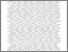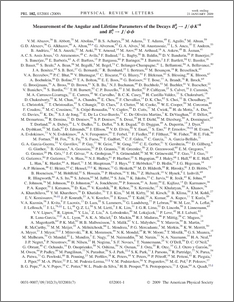# Measurement of the angular and lifetime parameters of the decays $B_{d}^{0}\to J/ψK^{*0}$ and $B_{s}^{0}\to J/ψφ$

Collaboration, D0 and Bertram, Iain and Borissov, Guennadi and Fox, Harald and Williams, Mark and Ratoff, Peter and Love, Peter and Rakitin, Alexander and Sopczak, Andre (2009) Measurement of the angular and lifetime parameters of the decays $B_{d}^{0}\to J/ψK^{*0}$ and $B_{s}^{0}\to J/ψφ$. Physical review letters, 102 (3). ISSN 1079-7114Preview
PDF
PhysRevLett.102.032001.pdf - Published Version

We present measurements of the linear polarization amplitudes and the strong relative phases that describe the flavor-untagged decays $B_{d}^{0}\to J/\psi K^{*0}$ and $B_{s}^{0}\to J/\psi\phi$ in the transversity basis. We also measure the mean lifetime $\bar{\tau}_{s}$ of the $B_{s}^{0}$ mass eigenstates and the lifetime ratio $\bar{\tau}_{s}/\tau_{d}$. The analyses are based on approximately 2.8 fb$^{-1}$ of data recorded with the D0 detector. From our measurements of the angular parameters we conclude that there is no evidence for a deviation from flavor SU(3) symmetry for these decays and that the factorization assumption is not valid for the $B_{d}^{0}\to J/\psi K^{*0}$ decay.View Item